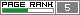Friday, March 17, 2006

Evolution Of An Optical Lens Through Evolution Strategy.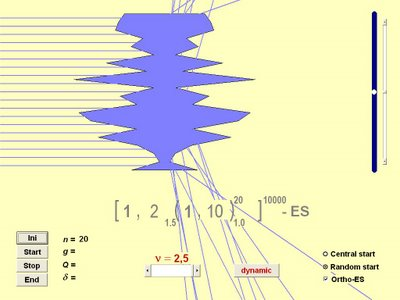Hallo!!

I was looking for interesting applications of Evolutionary Computation and I found the paper Evolution Strategy in Action 10 ES-Demonstrations (try here too for a PostScript file.)

In that paper there are 10 applications of Evolution Strategies, but I selected just one application: The Evolution of a Prism Lens (click here for a more detailed explanation and to simulate the Evolutionary Lens in a applet, where also there is another applet to test a Genetic Algorithm in the same problem).

Click here to download a small program (36 KB only) that simulate the Evolutionary Lens. In this program, you can determine and variate some parameters of the lens, of the objective function and also of the Evolution Strategy. That program uses a Nested Evolution Strategy.

The Evolution of a Prism Lens

By means of Evolution Strategy the shape of an initially cuboid light-transmissive body is to be changed in such a way, that parallel falling rays of light are refracted into a single point P on a projection surface.

The object

The light transmissive body consists of a pile of n prism which form a polygonal lens. Therefore the thickness of this lens can be altered at (n+1) locations. The refraction numbers of the prism and the surrounding medium are r2 and r1 respective. The proportion r2/r1 of the refraction numbers is adjustable between 0.2 and 5.0. If it is smaller than one, the lens may be formed by an inclusion of air in a body of glass.

A shorter explanation: The goal is to optimize the shape of lens in such a way, that parallel rays falling into the lens will focus in one single point. The shape is optimized by modifying the lens segments' thickness.

Here you can see some pictures of the Evolutionary Lens, in all start generation we have a random lens and the Evolution Strategy tries to optimize it (for more details about Lenses, click here or here):

1 - Biconvex Lens

Initial Lens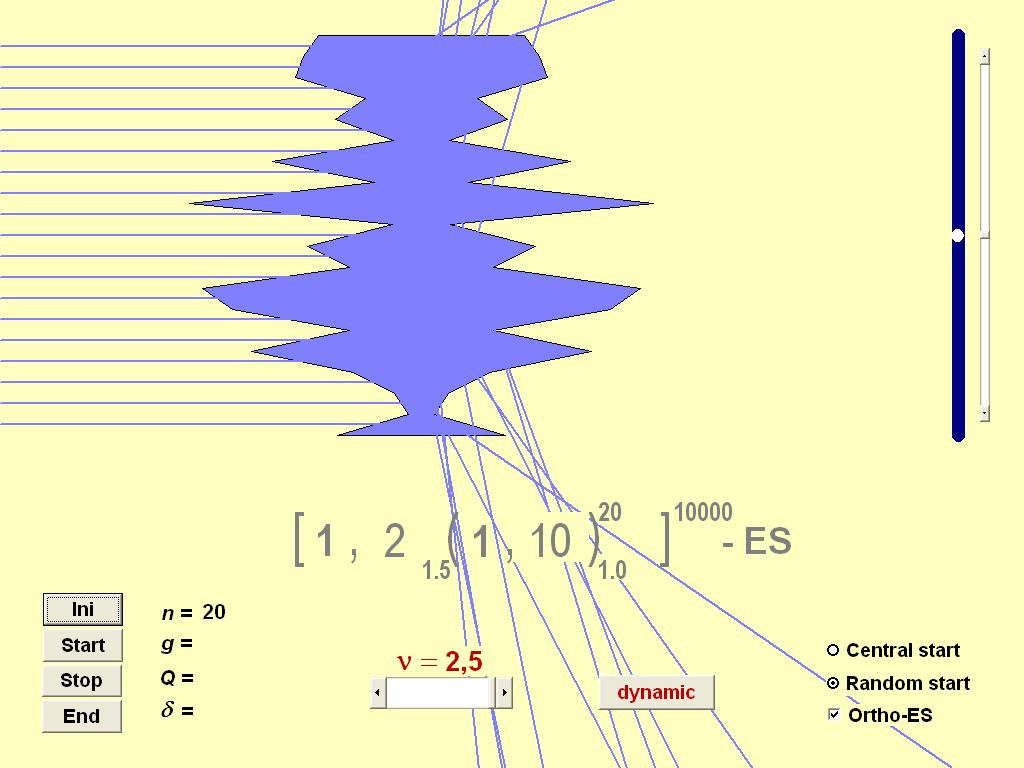Final Lens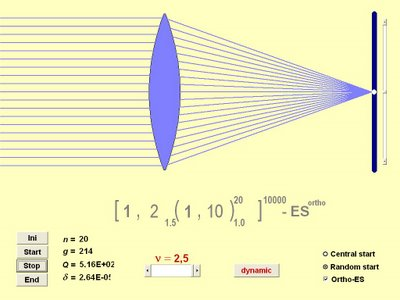2 - Biconcave Lens

Initial Lens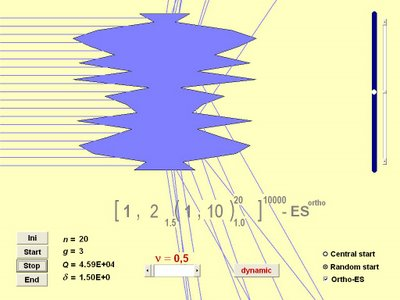Final Lens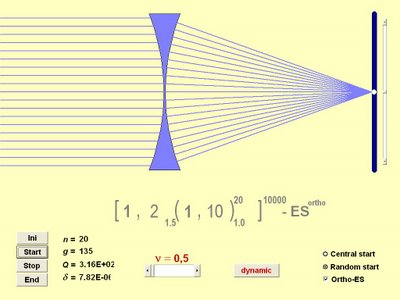3 - Dynamic Focal Point: In this one the focal point of the lens is dynamic, so its position changes along the generations and the Evolution Strategy needs to seek it.

Initial Lens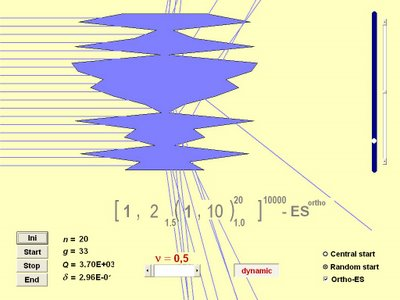Dynamic Focal Point Seeking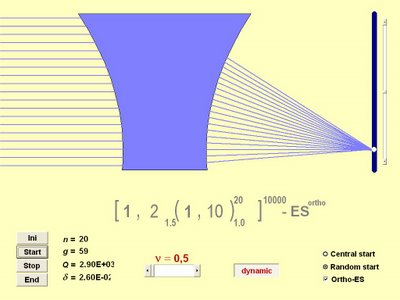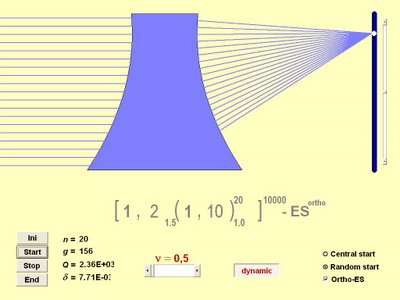In the Dynamic Focal Point Seeking I used a Biconcave Lens, but you can use a Biconvex with no problem.

Test the programs and have fun!! :D

Até Mais!!

Marcelo